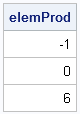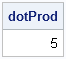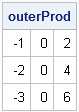For programmers who are learning the SAS/IML language, it is sometimes confusing that there are two kinds of multiplication operators, whereas in the SAS DATA step there is only scalar multiplication. This article describes the multiplication operators in the SAS/IML language and how to use them to perform common tasks such as the elementwise product, the dot product, and the outer product of vectors.

### Elementwise multiplication (#)

The elementwise multiplication operator (#) is used to perform element-by-element scalar multiplication. This operator is not part of the DATA step syntax. If you have two matrices of the same dimension, then u#v is the matrix whose ith element is the product of the ith elements of u and v. (This product is also known as the Hadamard product.) This is shown in the following PROC IML example:

```proc iml; u = { 1, 2, 3}; /* 3x1 column vector */ v = {-1, 0, 2}; /* 3x1 column vector */   elemProd = u#v; /* elementwise product (Hadamard product) */ print elemProd;```The elementwise multiplication operator can also be used in some situations in which u is a vector that has the same row or column dimension as v. See my article on how the SAS/IML language "knows what you want."

### True matrix multiplication (*)

The matrix multiplication operator (*) performs true matrix multiplication. Whereas the * operator is used for scalar multiplication in the DATA step, the operator is used for matrix multiplication in PROC IML. If u and v are any two matrices where the number of columns of u matches the number of rows of v, then the matrix product u*v is defined.

When u and v are vectors, matrix multiplication gets a special name. When a row vector is multiplied with a column vector, the result is a scalar and the operation is called the dot product (or inner product or scalar product). The following example uses the transpose operator (`) to create a row vector:

```dotProd = u`*v; /* dot product (scalar product, inner product) */ print dotProd;```There is an interesting connection between the elementwise product and the dot product of two vectors. The dot product of u and v is the same as the sum of the elements of the elementwise product: u`*v = sum(u#v).

Matrix multiplication is not commutative, so you get a different result if you multiply a column vector with a row vector. The result is a rank-1 matrix. This is called the outer product of two vectors. An example follows:

```outerProd = u*v`; /* outer product: column vec times row vec */ print outerProd;```### Other matrix products

The SAS/IML language supports other kinds of multiplication, including the direct product (or Kronecker product) and the horizontal direct product of matrices:

```dirProd = u`@v; /* direct product */ hdirProd = hdir(u,v); /* horizontal direct product */```

There are many special-purpose products that are not covered in this short article, but remember that you can always define your own SAS/IML function that compute any conceivable product. For example, in physics classes students use the "cross product" (also called the skew-symmetric product) to compute quantities that arise in electromagnetism. The following SAS/IML function implements the cross product computation:

```/* cross product (3D vectors only) */ start CrossProd(u, v); i231 = {2 3 1}; i312 = {3 1 2}; return( u[i231]#v[i312] - v[i231]#u[i312] ); finish;   uxv = CrossProd(u,v);```

Be aware that in statistics, the "cross product" often refers to the multiplication X`*X, where X is a data matrix. In this matrix product, the (i,j)th element of X`*X is the dot product of the ith and jth columns of X.

ShareDistinguished Researcher in Computational Statistics

Rick Wicklin, PhD, is a distinguished researcher in computational statistics at SAS and is a principal developer of SAS/IML software. His areas of expertise include computational statistics, simulation, statistical graphics, and modern methods in statistical data analysis. Rick is author of the books Statistical Programming with SAS/IML Software and Simulating Data with SAS.

1.Hi Ric,

Very useful article - thanks.

I think I found two minor errors. In the true matrix multiplication paragraph you wrote "...where the number of rows of u matches the number of columns of v,...". I think rows and columns should be the other way round if you multiply u*v.

Second, the HDIR function didn’t like the transposed first vector when I tried your example. hdir(u, v) worked for me (SAS 9.3).

•Thanks for the careful reading. I will correct the errors.

2.This is out of topic question.
I want to calculate summary statistics and want to save in a dataset.
Please let me know , how i can do this.
and also please provide small example.

thank you.

•You can ask questions like this at the SAS/IML Support Community.

3.I have a n*n matrix A and I would like to calculate A2=A*A , A3=A2*A, A4=A3*A ... A60=A59*A. Can any one help me writing a do loop to perform this task ? I have never used IML before. I do have a piece of code to complete the same task in R.

Thank you !

•I assume this is homework. Initialize the result matrix matrix (call it B) to the identity matrix. Then loop sixty times, each time multiplying the current value of B times A. You can use the expression A**60 to make sure you get the correct answer. For future questions, please post to the SAS Support Community for SAS/IML.

4.For two large vectors, will there be any performance advantages in using element wise product vs. make one vector into a diagonal matrix then do matrix multiplication?

u # v vs. diag(u) * v

•Yes. The elementwise product will be much faster, as discussed in the article "Never multiply with a large diagonal matrix."